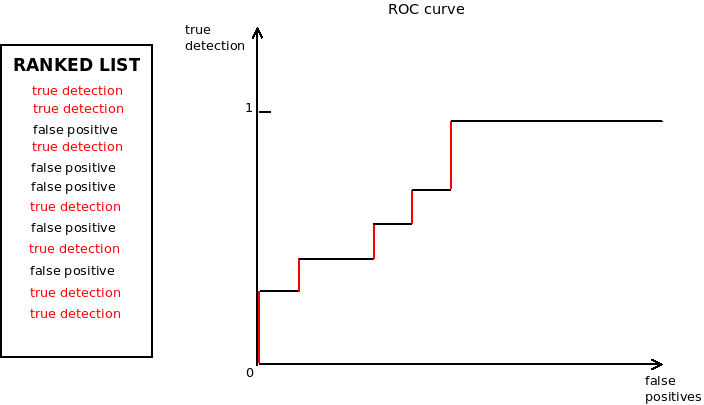# Generate ROC curve and compute AUC in Java

Sep 11, 2019 by Alexandre Croix | 3940 views

It is very common to implement a model to classify elements in different categories. A very important step in classification is the evaluation of the model efficiency.

The efficiency of a binary classification can be represented by the ROC curve (Receiver Operating Characteristic) and the AUC (Area Under the Curve).

## What is a ROC curve and the AUC?

The ROC is a graphical plot that illustrates the diagnostic ability of a binary classifier system as its discrimination threshold is varied. It is created by plotting the true positive rate (true detection) against the false positive rate (false alarm).The Area Under the Curve, as indicated by its name, is the area delimited by the ROC curve and the X axis.

It is easy to implement ROC generation and obviously, a lot of Java libraries can do that. However, most of these libraries are not dedicated to compute ROC and AUC and are bigger than necessary to perform a simple AUC computation.

The library java-roc is a small package that allows a simple way to generate ROC and compute AUC.

## Installation

The easiest way to install the package is to use Maven (check the latest version on https://mvnrepository.com/artifact/be.cylab/java-roc

``````<dependency>
<groupId>be.cylab</groupId>
<artifactId>java-roc</artifactId>
<version>0.0.4</version>
</dependency>``````

## Usage

The package uses a simple object ROC that takes 2 arguments:

• an array of double (score)
• an array of boolean (label)

The following example uses random scores:

``````double[] score = new double;
for (int i = 0; i < score.length; i++) {
score[i] = Math.random();
}
boolean[] true_alert = new boolean[] {
false,
true,
false,
true,
true,
false,
true,
true,
false,
false};
Roc roc = new Roc(score, true_alert);
System.out.println(roc.computeAUC());

List<RocCoordinates> roc_coordinates = roc.computeRocPointsAndGenerateCurve("Test.png");``````

The example generates a ROC curve that is stored in a file called Test.jpg.